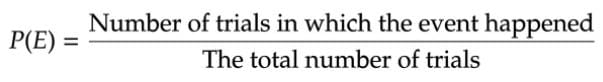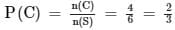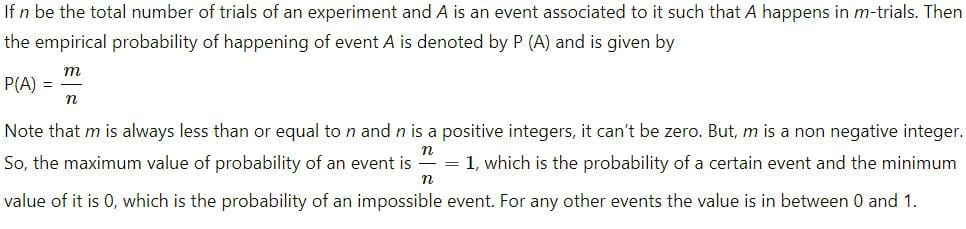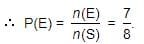# Test: Probability Experimental Approach

## 10 Questions MCQ Test Online MCQ Tests for Class 9 | Test: Probability Experimental Approach

Description
Attempt Test: Probability Experimental Approach | 10 questions in 10 minutes | Mock test for Class 9 preparation | Free important questions MCQ to study Online MCQ Tests for Class 9 for Class 9 Exam | Download free PDF with solutions
QUESTION: 1

### Empirical probability P (E) of an event happening is defined as:

Solution:

Definition of Experimental Probability: The experimental probability of the happening of an event is the ratio of the number of trials in which the event happened to the total number of trials.
The experimental probability of the occurrence of an event E is defined as:QUESTION: 2

### If the probability of winning the game is 0.7 then, what is the probability of losing it?

Solution:

The probability of anything is 1.
∵ It is given that the probability of winning the game is 0.7
Therefore, the probability of losing the game is
1 - 0.7 = 0.3

QUESTION: 3

### One card is drawn at random from a pack of 52 cards. What is the probability that the card drawn is either a red card or a king ?

Solution:

Clearly, n(S) = 52.
There are 26 red cards (including 2 kings ) and there are 2 more kings.
Let (E) be the event of getting either a red card or a king.
⇒ n(E) = 28
∴ P(E) = n(E) / n(S) = 28/52 = 7/13

QUESTION: 4

An unbiased dice is thrown. What is the probability of getting an even number or a multiple of 3?

Solution:

the event of getting a number that is either an even number or a multiple of 3.
C = {2,3,4,6}
n(C) = 4QUESTION: 5

The maximum probability of an event of a trial is:

Solution:QUESTION: 6

In an experiment, 100 drawing pins were dropped on a floor. 73 landed point up and the rest landed point down. A pin is selected at random and dropped. What is the probability that the pin will land point down?​

Solution:

⇒ Total number of pins = 100

⇒ Number of pins that landed pointed up = 73

⇒ The number of pins that landed pointed down would be = 100-73 = 27

⇒ P( the pin selected and dropped would be pointed down) = Number of pins that landed pointed down / total number of pins.

⇒ Probability = 27/100 = 0.27

QUESTION: 7

What is the probability that a leap year has 53 Sundays?

Solution:

In a leap year, there are 366 days.
∵ There are 52 weeks
i.e.52×7=364 days

⇒ The remaining 2 days may be {(Sun, Mon), (Mon, Tue), (Tue, Wed), (Wed, Thu),(Thu, Fri), (Fri, Sat), (Sat, Sun)}.

∴ P (53 Sundays ) = 2/7.

QUESTION: 8

In a simultaneous throw of a pair of dice, the probability of getting a sum more than 7 will be______.

Solution:

E ⟶ event of getting a sum more than 7

No. of favorable outcomes = 15 {(2, 6) , (3, 5) , (3, 6) , (4, 4) , (4, 5) , (4, 6) , (5, 3) , (5, 4) , (5, 5) , (5, 6) , (6, 2) , (6, 3) , (6, 4) , (6, 5) , (6, 6)}

Total no. of possible outcomes = 36

P(E) = 15/36 = 5/12

QUESTION: 9

Three unbiased coins are tossed. What is the probability of getting at most two heads?

Solution:

Here S = {TTT, TTH, THT, HTT, THH, HTH, HHT, HHH}

Let E = event of getting at most two heads.

Then E = {TTT, TTH, THT, HTT, THH, HTH, HHT}.QUESTION: 10

An event for an experiment is defined as:

Solution:

An event that cannot possibly happen has a probability of zero. If there is a chance that an event will happen, then its probability is between zero and 1.
Examples of Events: tossing a coin and it landing on heads.Use Code STAYHOME200 and get INR 200 additional OFF Use Coupon Code